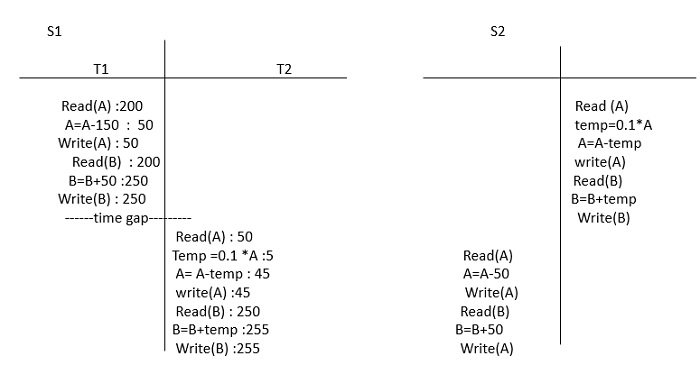# What are the different ways the transaction can be executed(DBMS)?

There are three possible ways in which a transaction can be executed. These are as follows −

• Serial execution − In serial execution, the second transaction can begin its execution only after the first transaction has completed. This is possible on a uniprocessor system.

• Parallel execution − In parallel execution, two transactions can start their execution exactly at the same instant of time. For this, we require more than one processor.

• Concurrent execution − In concurrent execution, execution of the second process can begin even before the process has completed its execution.

Let us consider two transactions T1 and T2. In concurrent execution, the CPU executes some instructions of transaction T1, then moves to the second transaction and executes its instructions for some time and again it comes back to the first transaction.

The cycle is repeated until all the instructions of both the transactions are executed. The process is called context switching.

The advantages of transaction in the database management system (DBMS) are as follows −

• Optimum utilization of resources.
• Average waiting time is less.
• Response time is shorter.
• Throughput is more (number of processors executed on one unit of time).

## Example

Given below is an example of the serial execution −

Let us consider two transactions T1 and T2 where T1 performs transfer Rs.150 from account A to account B and similarly T2 transfers 10% of balance from A to B.

## Solution

  T1                            T2
A=A-150                       temp =0.1 *A
Write(A)                      A=A-temp
B=B+temp
Write(B)

The order in which the instruction of transactions T1 and T2 are executed is called a Schedule. The possible serial schedules are as follows −

Let A= 200, B=200Schedule S1 and S2 keeps the database in consistent state.

In general if the system consists of n number of transactions, then we can generate n! number of valid serial schedules.